Select Page

# Maths 12 Science Relations and Functions CBSE Solutions for MCQ in English

Maths 12 Science Relations and Functions CBSE Solutions for MCQ in English to enable students to get Solutions in a narrative video format for the specific question.

Expert Teacher provides Maths 12 Science Relations and Functions CBSE Solutions for MCQ through Video Solutions in English language. This video solution will be useful for students to understand how to write an answer in exam in order to score more marks. This teacher uses a narrative style for a question from Relations and Functions not only to explain the proper method of answering question, but deriving right answer too.

Please find the question below and view the Solution in a narrative video format.

Question:

Solution Video in English:

You can select video Solutions from other languages also. Please check Solutions in ( Hindi )

## Similar Questions from CBSE, 12th Science, Maths, Relations and Functions

Question 1 : If f(x) = |x| and g(x) = | 5x – 2 |. Then, fog = _____. (View Answer Video)

Question 2 :  A function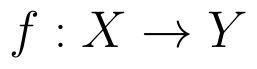is surjective if and only if , (View Answer Video)

Question 3 : The number of binary operations which can be defined on the set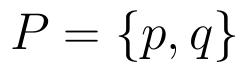is, (View Answer Video)

Question 4 : Number of binary operations on the set { a, b} are: (View Answer Video)

Question 5 : Letdefined as f(x) = x be an identity function. Then, (View Answer Video)

### Integrals

Question 1 : Evaluate :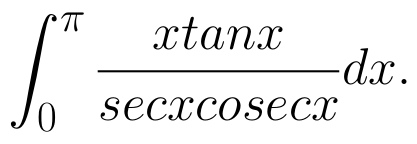(View Answer Video)

Question 2 : Find the integral of the function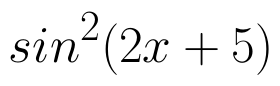. (View Answer Video)

Question 3 : Evaluate :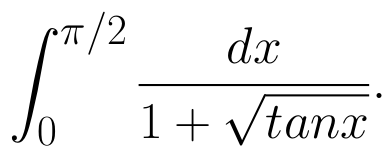(View Answer Video)

Question 4 : Find the integral of the function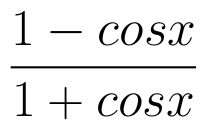. (View Answer Video)

Question 5 : Find :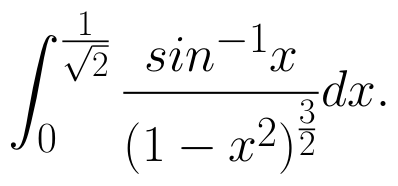(View Answer Video)

### Continuity and Differentiability

Question 1 : Differentiate the function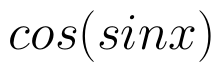with respect to x. (View Answer Video)

Question 2 : Differentiate the function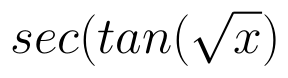with respect to x. (View Answer Video)

Question 3 : If x and y are connected parametrically by the equation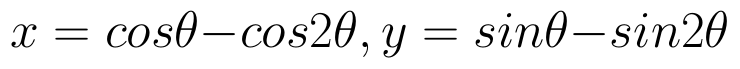, without eliminating the parameter, find. (View Answer Video)

Question 4 : Findfor the function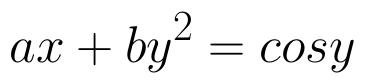. (View Answer Video)

Question 5 : Differentiate the functionwith respect to x. (View Answer Video)

### Application of Integrals

Question 1 : Find the area bounded by the curve y = sin x between x = 0 and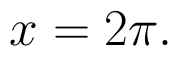(View Answer Video)

Question 2 : Using integration, find the area of the triangle formed by a positive x-axis and tangent and normal to the circle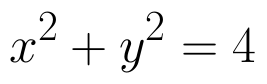at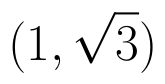. (View Answer Video)

Question 3 : Using integration, find the area bounded by the tangent to the curve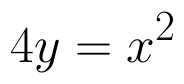at the point (2, 1) and the lines whose equations are x = 2y and x = 3y - 3. (View Answer Video)

Question 4 : Find the area enclosed by the parabola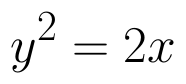and the line x - y = 4. (View Answer Video)

Question 5 : Using the method of integration, find the area of the region bounded by the lines 3x - y - 3 = 0, 2x + y - 12 = 0 and x -2y - 1 = 0. (View Answer Video)